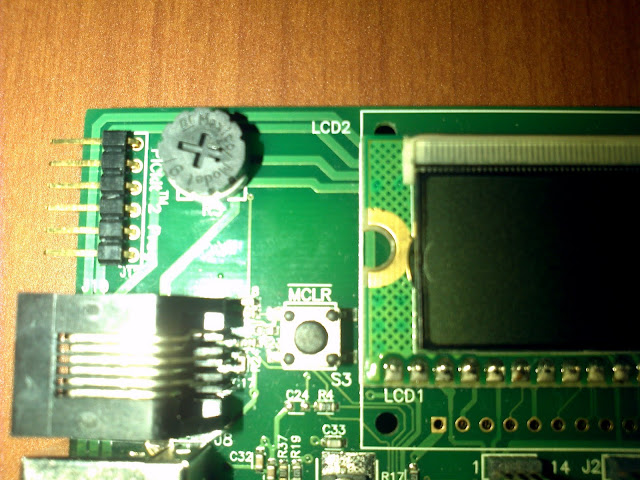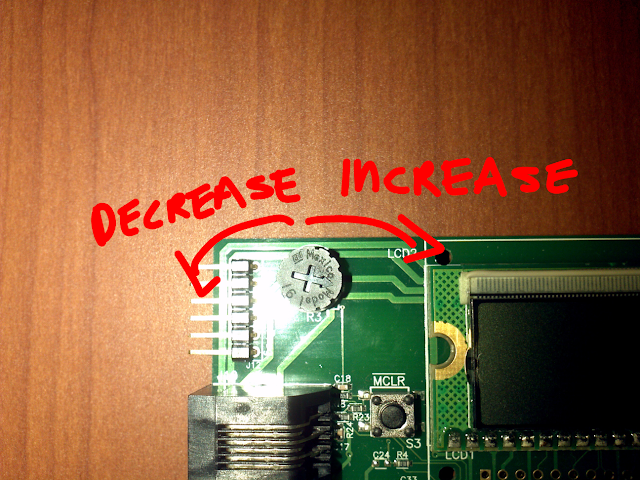# PIC18 Explorer Board Tutorial: Analog to Digital Conversion With the Potentiometer

by on June 6, 2011The potentiometer in a PIC18 Explorer Board

## The Program We’ll Be Writing

We will write a program that will read the voltage at the potentiometer and we will display that voltage using the LEDs in port D.

## The Potentiometer

The potentiometer is the wheel to the left of the LCD, it is connected to RA0. Turning the potentiometer to the right increases the voltage at RA0, turning it left decreases the voltage.turning it right increases input voltage, turning it left decreases it

The data we are reading is a binary representation of the voltage at the pot (RA0), more precisely it is a 10 bit binary number, actually split into two (uneven) sections: the most significant and least significant bits. We will only be using the most significant bits which will be eight ( the least significant bits are 2)

If all the bits are 1 we have the highest possible voltage ( ideally 5.0V) all bits zero will mean 0.0V (ideally).

```// example
0b00000000; // will be least voltage
0b11111111; // will be max voltage
```

any other binary number in between is a voltage between 0V and 5V.

## Configure The Ports

Since the pot is located at port A we will make port A an input; port D will be an output.

```	TRISA=0xff; // port A input
TRISD=0x00; // port D output
```

## Configuring The A/D Module

There are three bit sets you need to configure ADCON0, ADCON1 and ADCON2, each bit set has eight bits.

The first two most significant bits (from left to write) are unimplemented so we can leave them as zeros.

```	ADCON0=0b00
```

The next four let you choose the channel want to use, since the potentiometer is connected to AN0 we will make all the four bits zeros.

```	ADCON0=0b000000
```

The next bit let’s you start the analog to digital conversion, in other words when ever you give it a value of 1 it will start reading the voltage, we are not there yet so make a zero.

```	ADCON0=0b0000000
```

Lastly we want to turn on the analog to digital module

```	ADCON0=0b00000001;
```

You can read page 270 of the datasheet to see all the available options.

Similarly for ADCON1 one we have (from left to right) the first two bits which are unimplemented.

```ADCON1=0b00
```

The next two represent your reference voltage. I’ll be using AVDD and AVSS so my bits are all zeros

```ADCON1=0b0000
```

The next four bits let you select which ports you want to be analog and which digital. We can use several codes since we need AN0 to be analog and we are not worried about the rest, so I’ll be using all zeros

```ADCON1=0b00000000;
```

Check page 271 of the datasheet to see all options that are available.

This is the last bit set we need to configure before we start the conversion.

The first bit lets you select the justification of your result (left of right), we want left so ours is 0;

```ADCON2=0b0
```

The next bit is unimplemented so make it zero as well

```ADCON2=0b00
```

Next three bits are to set the time acquisition delay, there are several options (check page 272 of the datasheet) we want to go with 0 TAD so we have all zeros.

```ADCON2=0b00000
```

The last three bits set the oscillator, we are going with FOSC/32 so we need a two (in binary)

```ADCON2=0b00000010;
```

## Starting The Conversion and Reading the Result

Setting the GO_DONE bit of ADCON0 to 1 will start the conversion, GO_DONE will change to zero when the conversion is finished. The result of the conversion is store in ADRESH and ADRESL, most significant and least significant bits respectively.

So here is what we need

```ADCON0bits.GO_DONE=1; // starts conversion
PORTD=ADRESH; // turn on lights, this is outside of the while loop
```

## The Complete Code

Putting everything together we end up with this program.

```#include <p18f8722.h>

#pragma config OSC=HS // high speed oscillator
#pragma config WDT=OFF // watch dog off

void main()
{

TRISA=0xff; // port A input
TRISD=0x00; // port D output
PORTD=0x00; // port lights off
PORTA=0x00;

MEMCONbits.EBDIS=1; // make port d digital

// (00) unimplemented (0000) channel AN0 (0)don't start yet (1) turn on analog to digital module, see page 270

// (00) unimplemented (00) using AVDD and AVSS (0000) A/D port bits, see page 271